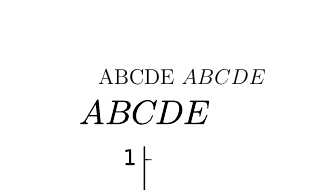# How to include PDF image from Sage into LaTeX document (scale issue)?

Hello!

I have a PDF illustration prepared in Sage:

p = plot(sin, 0, pi, fontsize=12, axes_labels=('ABCDE', ur'$ABCDE$'))
p.save('/tmp/sage1.pdf')


And the following LaTeX document:

\documentclass[12pt]{article}

\usepackage{graphicx}
\graphicspath{{/}}

\begin{document}
ABCDE $ABCDE$ \\
\includegraphics{sage1.pdf}
\end{document}


The problem is that the font size is inconsistent - is is clearly bigger in the picture than in document. I know about width parameter for includegraphics and other scaling option, but how to select the scale? Or, more generally, how to include PDF graphics to get exactly the same font size?edit retag close merge delete

Sort by » oldest newest most voted

You have to add axes_labels_size=1 to the arguments of plot:

p = plot(sin, 0, pi, fontsize=12, axes_labels=('ABCDE', ur'$ABCDE$'), axes_labels_size=1)


Indeed, axes_labels_size sets the relative size of the axes labels with respect to fontsize and its default value is 1.6.

more

You have two degrees of freedom here, one is to scale the figure directly in latex, e.g. \includegraphics[width=8cm]{sage1.pdf}, or change the font size in the sage document. To do this, you could type p.fontsize(10) or some appropriate number just before p.save(). Just type p. and press the tab key to see all possible commands that can be passed to your plot. Also, type p.save? in the worksheet to find out about other options (e.g. legend_font_size()). Hope this helps.

more